# SSAT Middle Level Math : How to add fractions

## Example Questions

### Example Question #32 : How To Add Fractions

Solve the equation: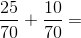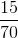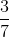Explanation:

When adding fractions that have the same denominator, only the numerators are added together. Add the numerators and keep the denominators: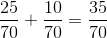This fraction can be reduced by dividing both the numerator and denominator by a common factor.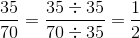Answer:### Example Question #33 : How To Add Fractions

Solve the equation: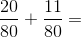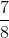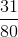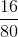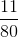Explanation:

When adding fractions that have the same denominator, only the numerators are added together. Add the numerators and keep the denominators: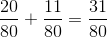Answer:### Example Question #34 : How To Add Fractions

Solve the equation: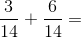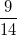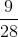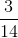Explanation:

When adding fractions that have the same denominator, add the numerators only. To solve add the numerators and keep the denominators the same: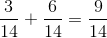Answer:### Example Question #35 : How To Add Fractions

Solve the equation: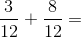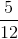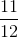Explanation:

When adding fractions that have the same denominator, only the top numbers are summed. Add the numerators and leave the denominators the same: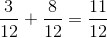Answer:### Example Question #36 : How To Add Fractions

Solve the equation: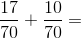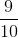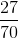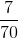Explanation:

When adding fractions that have the same denominator, only the top numbers are summed. Add the numerators and leave the denominators the same: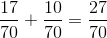Answer:### Example Question #331 : Fractions

Add: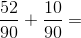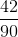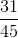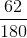Explanation:

Add the numerators and keep the denominators the same: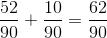Reduce the fraction: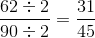### Example Question #332 : Fractions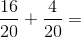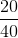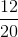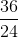Explanation: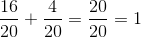### Example Question #333 : Fractions

Add: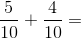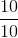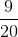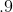Explanation:

Add the numerators and leave the denominators the same: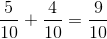Answer:### Example Question #334 : Fractions

Add: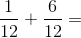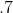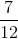Explanation:

Add the numerators and leave the denominators the same:Answer:### Example Question #335 : Fractions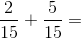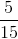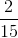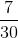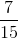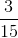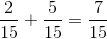Answer: